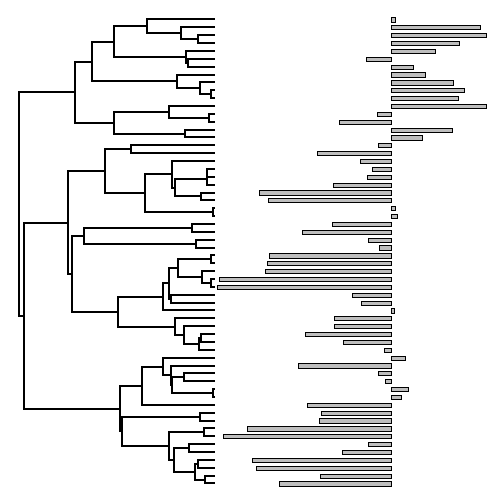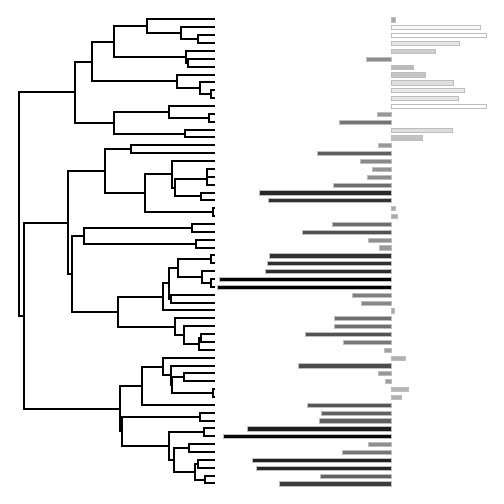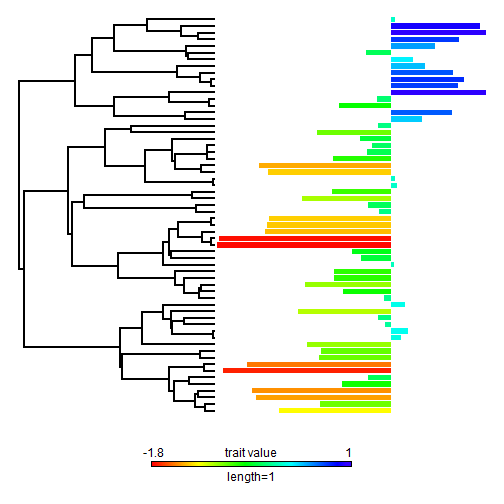## Sunday, October 25, 2015

### New features in phytools function plotTree.wBars

On the suggestion of Frederico Faleiro I just added two new features to the phytools plotting function `plotTree.wBars`.

First, the user can now set the bar colors. This can be a single color, or different colors for different bars. Second, the user can now set or turn off bar borders.

Here's a quick demo:

``````library(phytools)
tree<-pbtree(n=60,scale=1)
x<-fastBM(tree)
## basic method:
plotTree.wBars(tree,x,scale=0.5)
``````Now, greyscale by value:

``````col<-grey((x-min(x))/(max(x)-min(x)))
col<-setNames(col,names(x))
col[1:10] ## e.g.
``````
``````##       t44       t45       t31       t32       t23       t24       t42
## "#3B3B3B" "#626262" "#252525" "#212121" "#767676" "#8F8F8F" "#060606"
##       t43       t34       t35
## "#1D1D1D" "#606060" "#626262"
``````
``````plotTree.wBars(tree,x,col=col,scale=0.5,border="grey")
``````Rainbow by value (a little trickier):

``````## just one way to do this:
obj<-contMap(tree,x,plot=FALSE)
foo<-function(i,obj){
ind<-which(obj\$tree\$edge[,2]==i)
n<-names(obj\$tree\$maps[[ind]])[length(obj\$tree\$maps[[ind]])]
obj\$cols[n]
}
col<-setNames(sapply(1:Ntip(tree),foo,obj=obj),obj\$tree\$tip.label)
plotTree.wBars(tree,x,col=col,scale=0.5,border="transparent",width=0.8,
ylim=c(-10,Ntip(tree)))